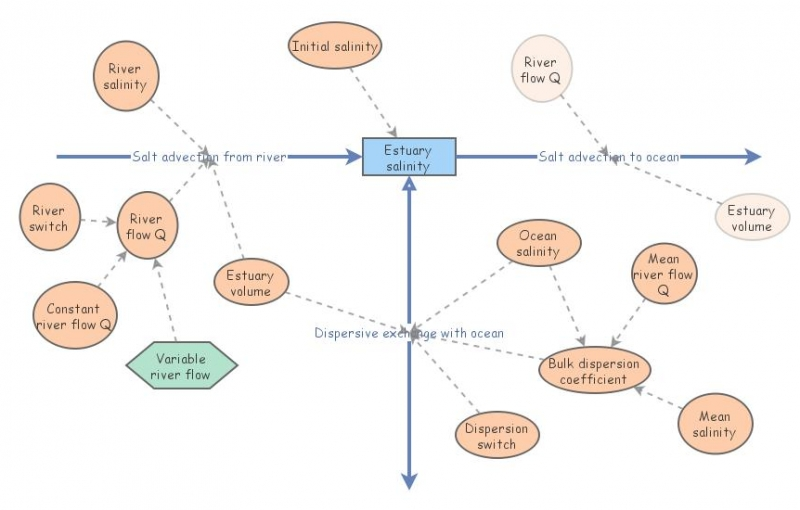Estuarine salinity 1D modelThis model implements the one-dimensional version of the advection-dispersion equation for an estuary. The equation is:

dS/dt = (1/A)d(QS)/dx - (1/A)d(EA)/dx(dS/dx) (Eq. 1)

Where S: salinity (or any other constituent such as chlorophyll or dissolved oxygen), (e.g. kg m-3); t: time (s); A: cross-sectional area (m2); Q: river flow (m3 s-1); x: length of box (m); E: dispersion coefficient (m2 s-1).

For a given length delta x, Adx = V, the box volume. For a set value of Q, the equation becomes:

VdS/dt = QdS - (d(EA)/dx) dS (Eq. 2)

EA/x, i.e. (m2 X m2) / (m s) = E(b), the bulk dispersion coefficient, units in m3 s-1, i.e. a flow, equivalent to Q

At steady state, dS/dt = 0, therefore we can rewrite Eq. 2 for one estuarine box as:

Q(Sr-Se)=E(b)r,e(Sr-Se)-E(b)e,s(Se-Ss) (Eq. 3)

Where Sr: river salinity (=0), Se: mean estuary salinity; Ss: mean ocean salinity

E(b)r,e: dispersion coefficient between river and estuary, and E(b)e,s: dispersion coefficient between the estuary and ocean.

By definition the value of E(b)r,e is zero, otherwise we are not at the head (upstream limit of salt intrusion) of the estuary. Likewise Sr is zero, otherwise we're not in the river. Therefore:

QSe=E(b)e,s(Se-Ss) (Eq. 4)